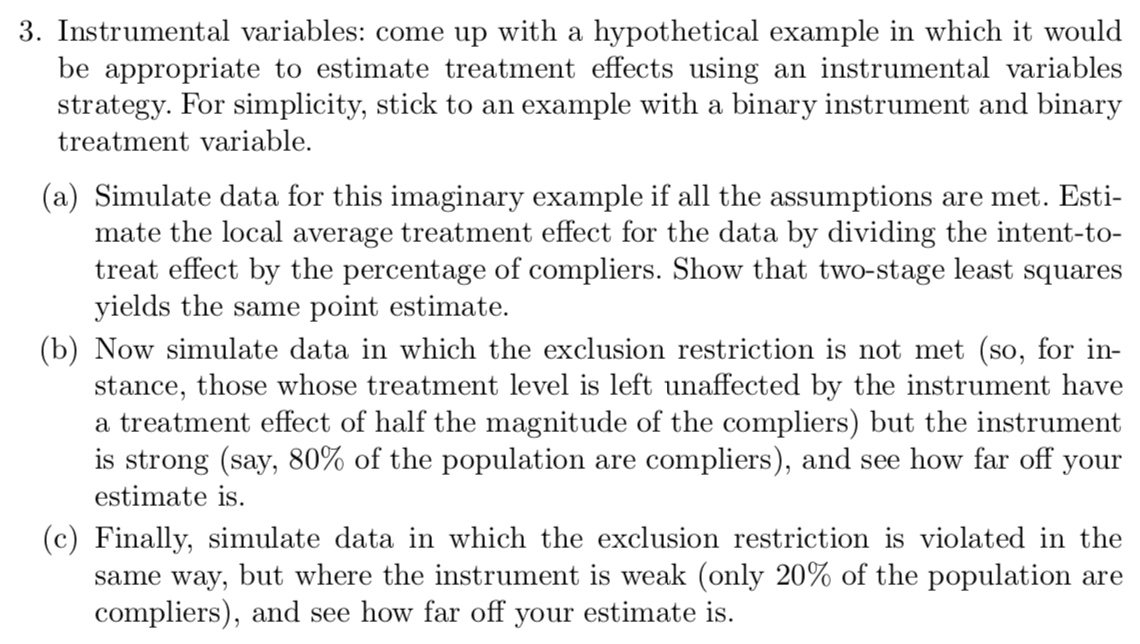## How to simulate an instrumental variables problem?

Edward Hearn writes:

In an effort to buttress my own understanding of multi-level methods, especially pertaining to those involving instrumental variables, I have been working the examples and the exercises in Jennifer Hill’s and your book.

I can find general answers at the Github repo for ARM examples, but for Chapter 10, Exercise 3 (simulating an IV regression to test assumptions using a binary treatment and instrument) and for the book examples, no code is given and I simply cannot figure out the solution.

I have no homework solutions to send. But maybe some blog commenters would like to help out?

Here’s the exercise:1.Samuel says:

When I’ve needed to simulate data from an IV model, I’ve had the best success by thinking about the IV model in terms of potential outcomes and principal strata. I’ll write out a.) what the principal strata are, b.) what my model for the potential outcomes within those principal strata is, and then c.) what my various assumptions say about the distribution of the principal strata and the parameters for the potential outcome models. Then I simulate the potential treatments and potential outcomes for the full sample, followed by simulating the instrument and constructing the observed data based on the instrument values.

This is especially straightforward in the case of a binary instrument and binary treatment variable, as there are then only 4 principal strata to worry about: the well-known Compliers, Defiers, Never Takers and Always Takers. To simulate from these groups, we just need to assign class probabilities to these four principal strata. At this point, we can start adding in model assumptions in terms of how we choose these probabilities. For instance, we can make the monotonicity assumption by assigning a probability of 0 to the Defier class. Then, we can think about a model for the conditional distribution of the potential outcomes within each of these principal strata. For instance, we may model Y(1) | Complier ~ N(mu_{C1}, sigma^2_{C1}) and Y(0) | Complier ~ N(mu_{C0}, sigma^2_{C0}). This tells us that the Complier Average Causal Effect is mu_{C1} – mu_{C0}. Here again we can add in assumptions through our choice of parameters. For instance, the exclusion restriction says that the instrument has no effect on the outcome except through the treatment actually received. In other words, the distribution of Y(1) | Never Taker is the same as the distribution of Y(0) | Never Taker, and similarly for the Always Takers.

By the way, this paper by Imbens and Rubin is a fantastic explanation of the binary treatment/binary instrument IV model, and shows how to approach IV models in terms of distributions of potential outcomes conditional on principal strata: https://projecteuclid.org/download/pdf_1/euclid.aos/1034276631

Here’s a short R script demonstrating this approach for the binary instrument/binary treatment IV model under the exclusion restriction:

###
n <- 100

# we'll assume there are only Never Takers and Compliers, with 80% of the population being Compliers

complier <- rbinom(n, 1, .8)

# we now generate potential outcomes Y(0) and Y(1) for each person in our sample.
# we'll use a normal model where for simplicity all variances are equal to 1.
# we'll also generate Y(0) and Y(1) separately.
# correlation between potential outcomes can be incorporated by simulating
# from a bivariate normal distribution.

Y0 <- Y1 <- rep(NA, n)

# simulating potential outcomes for compliers.
# we'll make the CACE equal to 0.5.

Y0[complier == 1] <- rnorm(sum(complier), mean = 1, sd = 1)
Y1[complier == 1] <- rnorm(sum(complier), mean = 1.5, sd = 1)

# simulating potential outcomes for never takers.
# under the exclusion restriction, Y(0) and Y(1) have the same distribution for never takers

Y0[complier == 0] <- rnorm(n – sum(complier), mean = 1, sd = 1)
Y1[complier == 0] <- rnorm(n – sum(complier), mean = 1, sd = 1)

# simulate a randomized instrument

Z <- rbinom(n, 1, .5)

# construct observed data

# observed treatment is 0 if Z = 0.
# if Z = 1, observed treatment is 0 for never takers and 1 for compliers

treatment_observed <- 0 + Z*complier

Y_observed <- (1 – Z)*Y0 + Z*Y1
###

2.Anon says:

Try asking at https://stats.stackexchange.com/, including what you’ve tried so far?

3.Z says:

There’s a mistake in part (b). Compliers having a different treatment effect than non-compliers is not a violation of the exclusion restriction.

•Jennifer Hill says:

Part (b) isn’t saying that exclusion is violated because the noncompliers have a different treatment effect than compliers. It’s saying that exclusion is violated because they have a non-zero effect of the instrument. Setting the effect to half that of the compliers was just a way of making that non-zero effect concrete. But I agree that the wording is sloppy (particularly use of the word treatment). I have a better version that I used in my causal inference course last year that I’ll be adding to the new book this week! That one has been beta-tested by students so should be more clear.

•Andrew says:

Thanks, coauthor!